# Dissipative function

(diff) ← Older revision | Latest revision (diff) | Newer revision → (diff)

dissipation function

A function used to take into account the effect of the forces of viscous friction on the motion of a mechanical system. The dissipation function describes the rate of decrease of the mechanical energy of the system; it is also used, more generally, to allow for the transition of energy of ordered motion to energy of disordered motion (ultimately to thermal energy).

The dissipation function for an isotropic medium, referred to unit volume, has the form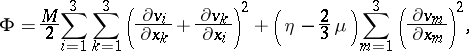where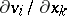are the components of the tensor of the deformation rates andandare the viscosity coefficients which describe the viscosity during motion and the viscosity during volume expansion, respectively.

The equation of change of entropy in a viscous medium has the form: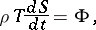whereis the specific entropy,is the density andis the temperature of the liquid.

The dissipation function is a characterization of the viscous forces during the motion of a continuous medium. The equation of motion of a viscous medium iswhere the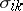are the components of the friction-less part of the stress tensor, theare the components of the "viscous" part of the stress tensor, and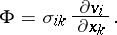The dissipation function is employed to allow for the effect of the resistance to small vibrations of the system around its equilibrium position; to study the damping of vibrations in an elastic medium; to allow for heat losses during the damping of electric current vibrations in circuit systems; etc.

How to Cite This Entry:
Dissipative function. Encyclopedia of Mathematics. URL: http://encyclopediaofmath.org/index.php?title=Dissipative_function&oldid=15366
This article was adapted from an original article by V.A. Dorodnitsyn (originator), which appeared in Encyclopedia of Mathematics - ISBN 1402006098. See original article